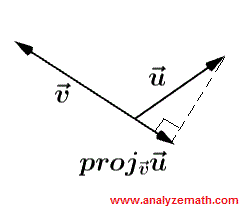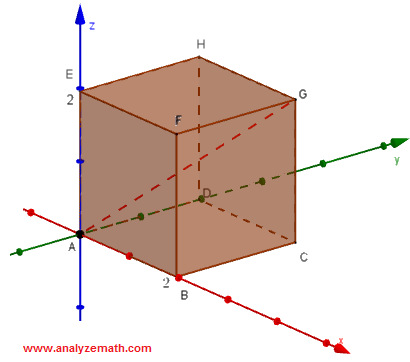# Solutions to Questions on Scalar and Cross Products for 3D Vectors

Detailed solutions to questions on Scalar and Cross Products of 3D Vectors are presented.

Answer with Detailed Solutions to Questions on Scalar and Cross Products of 3D Vectors.

1) Calculate $\vec{u} \cdot (\vec{u} \times \vec{v})$ given that $\vec{u} = \lt a,b,c \gt$ and $\vec{v} = \lt d,e,f \gt$.
Solution
The cross product $\vec{u} \times \vec{v}$ is a vector perpendicular to both vectors $\vec{u}$ and $\vec{v}$. Hence the dot product of two perpendicular vectors $\vec{u}$ and $\vec{u} \times \vec{v}$ is equal to 0.
$\vec{u} \cdot (\vec{u} \times \vec{v}) = 0$

2) Find $k$ so that vectors $\vec{u} = \lt -2,-k,1 \gt$ and $\vec{v} = <8,-2,-3>$ are perpendicular
Solution
If two vectors $\vec{u}$ and $\vec{v}$ are perpendicular then their dot product is equal to 0. Hence
$\vec{u} \cdot \vec{v} = \lt -2,-k,1 \gt \cdot <8,-2,-3> = 0$
Expand the dot product to obtain the equation.
$(-2)(8)+(-k)(-2)+(1)(-3) = 0$
Simplify and solve for $k$.
$-16 + 2 k - 3 = 0$
$k = 19/2$

3)Find $k$ so that the vectors $\vec{u} = \lt -3,2,-2 \gt$, $\vec{v} = <2,1,k>$ and $\vec{w} = <-1,3,-5>$ are on the same plane (or coplanar)?
Solution
Any two vectors are on the same plane (or coplanar). If a third vector is on this plane, the volume of the parallelepiped (see formula in Scalar and Cross Products of 3D Vectors ) formed by the 3 vectors is equal to 0. Hence the condition for any 3 (non zero) vectors to be coplanar is
$\vec{u} \cdot (\vec{v} \times \vec{w} ) = 0$
$\vec{u} \cdot (\vec{v} \times \vec{w} )$ is called the scalar triple product and is given by.
$\vec{u} \cdot (\vec{v} \times \vec{w} ) = det \begin{bmatrix} u_x & u_y & u_z \\ v_x& v_y & v_z \\ w_x& w_y & w_z \end{bmatrix}$
where $u_x, u_y, u_z, v_x...$ are the components of the three vectors.
We now substitute the components and calculate the determinant.
$\vec{u} \cdot (\vec{v} \times \vec{w} ) = det \begin{bmatrix} -3 & 2 & -2 \\ 2 & 1 & k \\ -1 & 3 & -5 \end{bmatrix}$ = -3(-5 - 3k) - 2(-10 +k) -2(6 + 1) = 21+7k
For the three vectors to be on the same plane, the determinant found above must be zero. Hence.
$21+7k = 0$
Solve the above equation for $k$ to obtain.
$k = -3$
Below are shown the three vectors on the same plane.4) Find angle $\theta$ between the vectors $\vec{u} = \lt 2,0,1 \gt$ and $\vec{v} = <8,-2,-3>$.
Solution
Use the definition of the scalar product of two vectors $\vec{u}$ and $\vec{v}$ and its expression using the vector components.
Definition: $\vec{u} \cdot \vec{v} = ||\vec{u}|| || \vec{v} || \cos \theta$ , θ is the angle between the two vectors.
The scalar product is also given by.
$\vec{u} \cdot \vec{v} = u_x v_x + u_y v_y + u_z v_z$ , where $u_x, v_x, u_y, ...$ are the components of the vectors $\vec{u}$ and $\vec{v}$.
Let us calculate the magnitudes $||\vec{u}||$ and $|| \vec{v} ||$ .
$||\vec{u}|| = \sqrt{2^2+0^2+1^2} = \sqrt{5}$
$||\vec{v}|| = \sqrt{8^2+(-2)^2+(-3)^2} = \sqrt{77}$
We now use the components to find the scalar product.
$\vec{u} \cdot \vec{v} = (2)(8)+(0)(-2)+(1)(-3) = 13$
We now use the definition to find angle θ.
$\vec{u} \cdot \vec{v} = ||\vec{u}|| || \vec{v} || \cos \theta$
$\cos \theta = \dfrac{\vec{u} \cdot \vec{v}}{||\vec{u}|| || \vec{v} ||} = \dfrac{13}{\sqrt{5}\sqrt{77}}$
$\theta = \arccos \dfrac{13}{\sqrt{5}\sqrt{77}} = 48.5^{\circ}$
5) Find the vector projection of $\vec{u} = \lt -1,-1,1 \gt$ onto $\vec{v} = <2,1,1>$.
Solution
The vector projection of of $\vec{u}$ on $\vec{v}$ is given by (see formula in Scalar and Cross Products of 3D Vectors ):
$\text{proj}_{\vec{v}}\vec{u} = \dfrac{\vec{u}\cdot\vec{v}}{||v||^2} \vec{v}$
$= \dfrac{ \lt -1,-1,1 \gt \cdot <2,1,1>}{2^2+1^2+1^2} <2,1,1> = \dfrac{(-1)(2)+(-1)(1)+(1)(1)}{6} <2,1,1>$
$= -\dfrac{2}{6} <2,1,1> = <-2/3,-1/3,-1/3>$
Vectors $\vec{u}$ , $\vec{v}$ and $\text{proj}_{\vec{v}}\vec{u}$ are shown below.6) Find that $k$ so that the points $A(-1,2,k)$, $B(-3,6,3)$ and $C(1,3,6)$ are the vertices of a right triangle with a right angle at $A$.
Solution
For triangle ABC to be right at A, the vectors $\vec{AB}$ and $\vec{AC}$ has to be perpendicular and therefore their scalar product is equal to 0. We start by calculating the components of the vectors.
$\vec{AB} = <-2,4,3-k>$
$\vec{AC} = <2,1,6-k>$
Scalar product of $\vec{AB}$ and $\vec{AC}$ has to be zero.
$<-2,4,3-k> \cdot <2,1,6-k> = 0$
$-4 + 4 + (3-k)(6-k) = 0$
Simplify and solve for k.
Two solutions: $k = 3$ and $k = 6$
7) Given vector $\vec{v} = \lt 3,-1,-2 \gt$, find vector $\vec{u}$ such that $\vec{v} \times \vec{u} = <4,2,5>$ and $||\vec{u}|| = 3$
Solution
Let a, b and c be the components of vector $\vec{u}$. Hence
$\vec{v} \times \vec{u} = {\begin{vmatrix}\vec{i}& \vec{j} &\vec{k} \\ 3 & -1 & -2 \\ a & b & c \end{vmatrix}}$
$= {\begin{vmatrix} -1 & -2 \\ b & c \end{vmatrix}} \vec{i} - {\begin{vmatrix}3 & -2\\ a & c\end{vmatrix}} \vec{j} + {\begin{vmatrix}3 & -1\\ a& b\end{vmatrix}} \vec{k} = (-c+2b)\vec{i} + (-2a-3c)\vec{j} + (3b+a)\vec{k}$
We now write that the components of $\vec{v} \times \vec{u}$ and $<4,2,5>$ are equal as given above. Hence
$-c+2b = 4$
$-2a-3c = 2$
$3b+a = 5$
Note that the equations in the above system are not independent ( add - 3 times the first equation -c+2b = 4 and the second equation -2a-3c = 2 and you will obtain the an equation equivalent to the third equation 3b+a = 5) and therefore it has many solutions. Let a = t and use second equation to find c.
-2t - 3c = 2
c = (2 + 2t) / (-3)
Let a = t again and use third equation to find b.
3b + t = 5
b = (5 - t) / 3
We now use the condition $||\vec{u}|| = 3$ to write the equation.
$\sqrt{a^2+b^2+c^2} = 3$
Square both side of the above equation and substitute a, b and c by their expressions in terms of t.
$t^2+((5 - t) / 3)^2+((2 + 2t) / (-3))^2 = 9$
Multiply all terms of the equation by 9 and simplify.
$9 t^2+(5 - t)^2+(2 + 2t)^2 = 81$
Expand the above to obtain a quadratic equation and solve it to find
t = 2 and t = -13/7
Hence two solutions for vector $\vec{u}$.
for t = 2 : $\vec{u_1} = < t , (5 - t)/3 , (2 + 2t)/(-3) > = <2 , 1, -2>$
for t = -13/7 : $\vec{u_2} = < t , (5 - t)/3 , (2 + 2t)/(-3) > = <-13/7 , 16/7 , 4/7>$
8) Points A, B, C and D forms a parallelogram.
a)Find the coordinates of point D.
b)Find the area of a parallelogram.Solution
a) Let a, b and c be the coordinates of point D and determine the components of vectors $\vec{AB}$ and $\vec{DC}$.
$\vec{AB} = <2 - 4 , 2 - 6 , 4 - 2 > = <-2 ,-4 ,2>$
$\vec{DC} = <-2 - a , -3 - b , 1 - c>$
For A, B, C and D to form a parallelogram, vectors $\vec{AB}$ and $\vec{DC}$ have to be equal (equivalent). Hence the vector equation
$<-2 , -4 , 2 > = <-2 - a , -3 - b , 1 - c>$
Which gives 3 algebraic equations.
-2 - a = -2
-3 - b = -4
1 - c = 2
Which gives the coordinates of point D.
D(0, 1 , -1)
b) The area A of the parallelogram is given by (see formula in Scalar and Cross Products of 3D Vectors )
A = $|| \vec{AB} \times \vec{BC} ||$
Let us first calculate the cross product,
$\vec{AB} \times \vec{BC} = {\begin{vmatrix}\vec{i}& \vec{j} &\vec{k} \\ -2 & -4 & 2 \\ -4 & -5 & -3 \end{vmatrix}} = {\begin{vmatrix} -4 & 2 \\ -5 & -3 \end{vmatrix}} \vec{i} - {\begin{vmatrix}-2 & 2\\ -4 & -3\end{vmatrix}} \vec{j} + {\begin{vmatrix}-2 & -4\\ -4& -5\end{vmatrix}} \vec{k} = 22\vec{i} + 14\vec{j} -6\vec{k}$
then its magnitude which is the area A.
A = $||\vec{AB} \times \vec{BC}|| = \sqrt{22^2+14^2+(-6)^2} = 2\sqrt{179}$
9) In the cube below find the angle between the diagonals AG and BH.Solution
We first find the components of the vectors $\vec{AG}$ and $\vec{BH}$.
$\vec{AG} = <2,2,2>$
$\vec{BH} = <-2,2,2>$
Using the dot product, with θ being the angle between the vectors $\vec{AG}$ and $\vec{BH}$, we have (see question 5 above).
$\cos \theta = \dfrac{\vec{AG} \cdot \vec{BG}}{||\vec{AG}|| || \vec{BH} ||} = \dfrac{2\cdot (-2)+2\cdot2+2\cdot2}{\sqrt{2^2+2^2+2^2}\sqrt{(-2)^2+2^2+2^2}} = \dfrac{1}{3}$
$\theta = \arccos \dfrac{1}{3} \approx 70.5^{\circ}$
10) Find a vector that is orthogonal to the plane containing the points A(1,2,-3), B(0,-2,1) and C(-2,0,1).
Solution
A vector $\vec{v}$ that is orthogonal to the plane defined above could be given by the cross product of any two distinct vectors in the plane. Hence.
$\vec{v} = \vec{AB} \times \vec{AC} = {\begin{vmatrix}\vec{i}& \vec{j} &\vec{k} \\ -1 & -4 & 4 \\ -3 & -2 & 4 \end{vmatrix}} = {\begin{vmatrix} -4 & 4 \\ -2 & 4 \end{vmatrix}} \vec{i} - {\begin{vmatrix}-1 & 4\\ -3 & 4\end{vmatrix}} \vec{j} + {\begin{vmatrix}-1 & -4\\ -3& -2\end{vmatrix}} \vec{k} = -8\vec{i} - 8\vec{j} -10\vec{k}$
Note that there are an infinite number of solutions.
11) Find the area of the triangle whose vertices are the points A(1,0,-3), B(1,-2,0) and C(0,2,1).
Solution
The area $A_t$ of a triangle is given by half the magnitude of the cross product of any two vectors made by the sides of the triangle. Hence
$A_t = \vec{AB} \times \vec{AC} = {\begin{vmatrix}\vec{i}& \vec{j} &\vec{k} \\ 0 & -2 & 3 \\ -1 & 2 & 4 \end{vmatrix}} = {\begin{vmatrix} -2 & 3 \\ 2 & 4 \end{vmatrix}} \vec{i} - {\begin{vmatrix}0 & 3\\ -1 & 4\end{vmatrix}} \vec{j} + {\begin{vmatrix}0 & -2\\ -1& 2\end{vmatrix}} \vec{k} = -14\vec{i} - 3\vec{j} -2\vec{k}$
$A_t = (1/2) || \vec{AB} \times \vec{AC} || = \sqrt{(-14)^2 + (-3)^2 + (-2)^2} = (1/2) \sqrt{209}$ unit
2
12)Find the volume of the parallelepiped shown below.Solution
The volume V of the parallelepiped is given by
V $= |\vec{u}\cdot (\vec{v} \times \vec{w})| = | \vec{v}\cdot (\vec{w} \times \vec{u})| = | \vec{w}\cdot (\vec{v} \times \vec{u})|$
Let us first find the components of the vectors $\vec{u}$, $\vec{v}$ and $\vec{w}$.
$\vec{u} = <-3,0,7>$
$\vec{v} = <-8,0,0>$
$\vec{w} = <0,-9,0>$
$\vec{u}\cdot (\vec{v} \times \vec{w})= <-3 , 0 , 7> \cdot {\begin{vmatrix}\vec{i}& \vec{j} &\vec{k} \\ -8 & 0 & 0 \\ 0 & -9 & 0 \end{vmatrix}}$
$= <-3 , 0 , 7> \cdot \left\{ {\begin{vmatrix} 0 & 0 \\ -9 & 0 \end{vmatrix}} \vec{i} - {\begin{vmatrix}-8 & 0\\ 0 & 0\end{vmatrix}} \vec{j} + {\begin{vmatrix}-8 & 0\\ 0 & -9\end{vmatrix}} \vec{k} \right\}$
$= <-3 , 0 , 7> \cdot <0 , 0 , 72> = 0 + 0 + 7\cdot72 = 504$
The volume is
$V = |504| = 504$ unit
3